## Java基础小白入门教程-----百知教育java基础学习1---胡鑫喆_百知教育java第一阶段考试题_wei198621的博客-程序员宅基地

Java基础小白入门教程（胡大大出品，彩蛋请自寻）

https://www.bilibili.com/video/BV1wE411V7Zo?from=search&seid=3851115456081760023

# 视频列表

P1-Java历史 29:40
P2-Java特点 38:43
P3-Java执行机制 34:27
P4-Java的HelloWorld 23:55
P5-环境变量的作用 33:01
P6-package和import语句 48:05
P7-学习方法介绍-费曼学习法 42:48
P8-编码规范-1 26:59
P9-编码规范-2 31:11
P10-计算机中的二进制 1:11:43
P11-变量和数据类型-1 51:27
P12-变量和数据类型-2 33:32
P13-数据类型的转换 28:24
P14-运算符1 1:00:23
P15-运算符2 25:37
P16-条件分支流程 51:00
P17-循环流程 55:35
P18-循环习题课 1:26:37
P19-函数的基本语法 45:31
P20-函数的参数和返回值 55:12
P21-函数的作用 43:04
P22-栈空间结构 30:04
P23-函数的递归 1:10:42
P24-函数习题课 30:43
P25-数组的基本语法 37:42
P26-数组的内存结构 1:19:44
P27-二维数组 29:45
P28-排序算法 44:18
P29-数组习题课-1 18:37
P30-数组习题课-2 1:29:20

# 1编译运行基础知识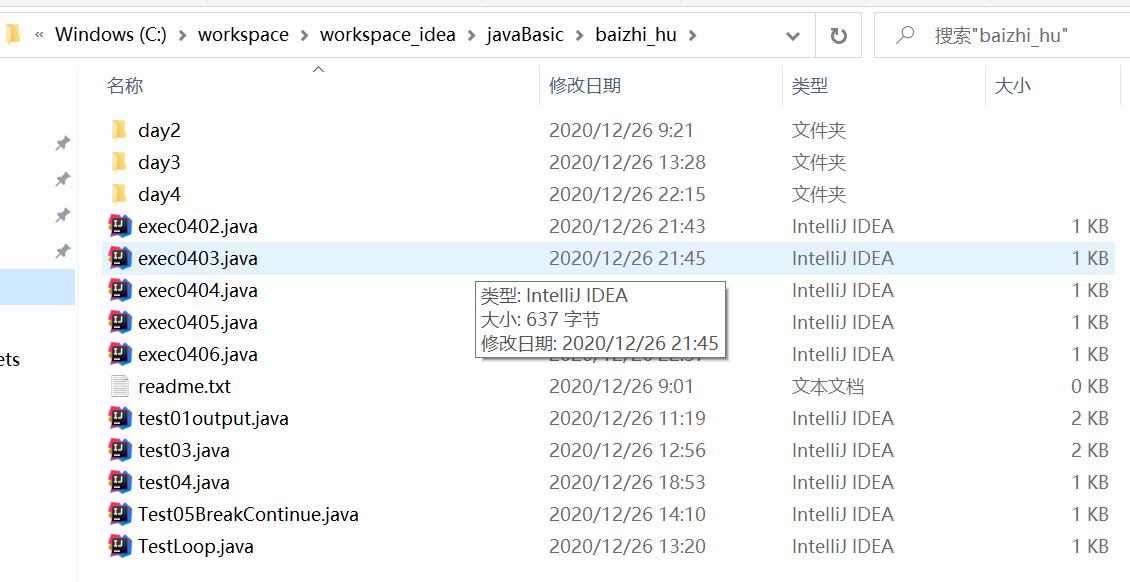C:\workspace\workspace_idea\javaBasic\baizhi_hu>javac -d . *.java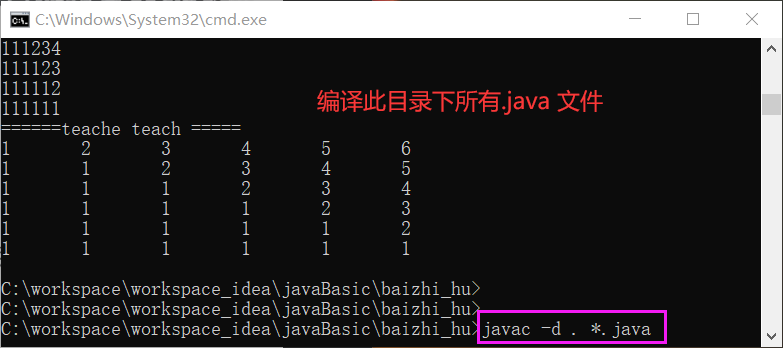C:\workspace\workspace_idea\javaBasic\baizhi_hu>java day4.exec0406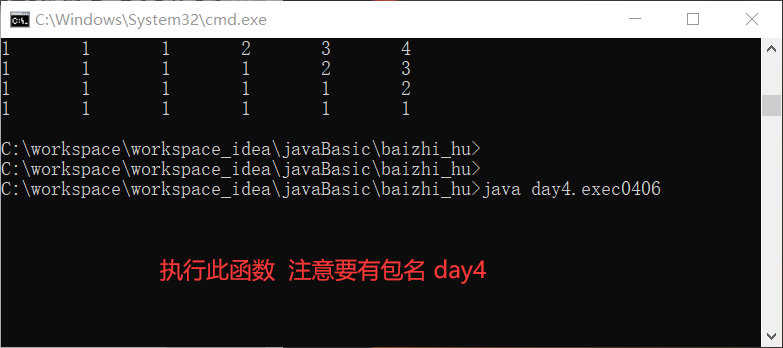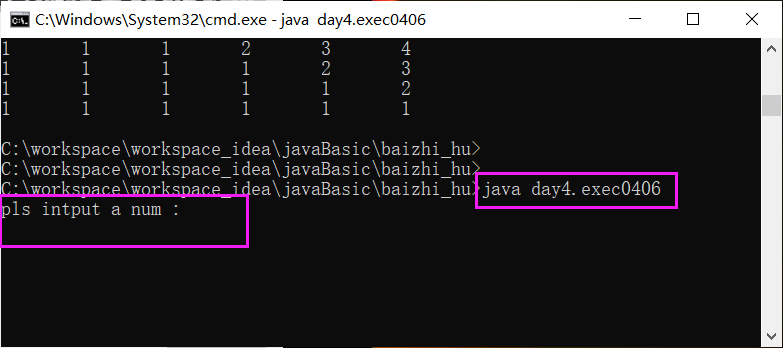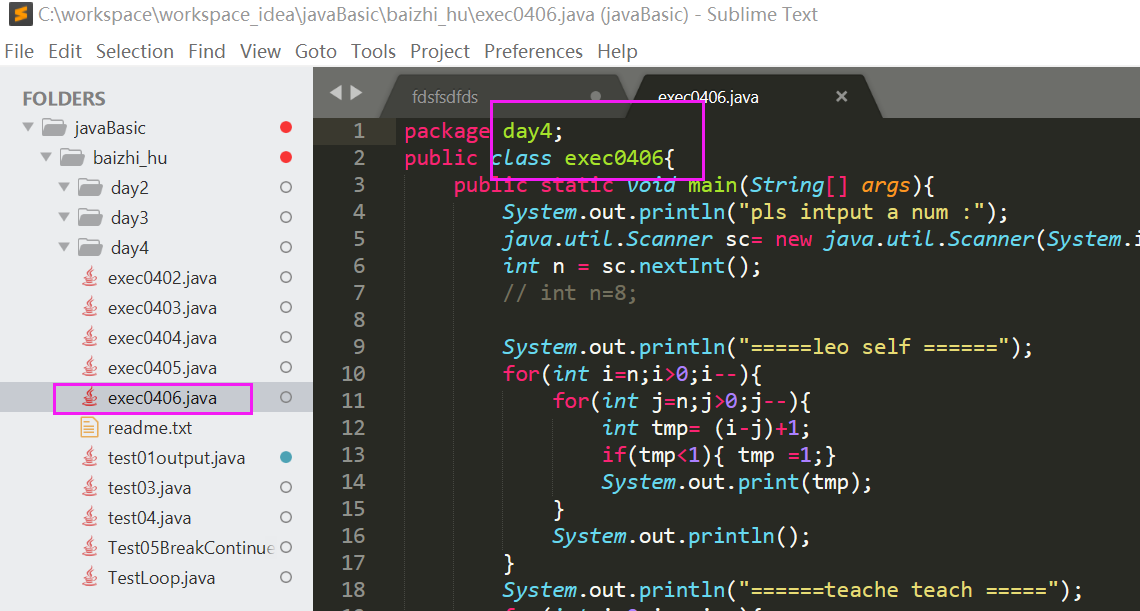# part1 基础

## P1-Java历史 29:40

``````1995 年   sun 诞生于
1998     JDK1.2 成熟 稳定
2004     JDK 1.5  复杂
2009      java 被oracle 收购
2014     JDK 1.8  Oracle改造版本
2018     JavaEE  --> JarkataEE
``````

JAVA 没有指针
TIOBE 排行第一

## P3-Java执行机制 34:27

1. 编译： 源代码 ----------> 机器码 （计算机直接执行的代码）
编译器
2. 解释 源代码 -----------> 逐行翻译为机器码并运行
解释器
3. Java 运行机制 先编译 后解释
源代码.java ---------> 字节码.class --------> 逐行翻译为机器码并运行
编译器 解释器
``````JVM   虚拟机    java virtual machine
JRE   运行环境  java Runtime Environment  （JVM+解释器）
JDK   开发环境  Java Development Kit   (JRE+类库+开发工具包)
``````

## P4-Java的HelloWorld 23:55

``````环境变量：
JAVA_HOME=JDK安装路径
Path= JAVA_HOME\bin
CLASSPATH=.

javac  a.java      ---- 编译java(会看到生成了一个Hello.class文件)
java Hello         --- java  准备运行的类的类名（不带扩展名.class 大小写敏感）
``````

## P5-环境变量的作用 33:01

``````环境变量
JAVA_HOME = JKD 安装路径     让其他需要java的程序使用此变量
Path = JAVA_HOME\bin         在任何路径下都可以运行java命令
CLASSPATH=.                   类路径  提示虚拟机到哪里找字节码文件
``````

## P6-package和import语句 48:05

``````类  公开类的类名必须与文件名一致，包括大小写一致
私有类就没有此限制
所以，一个源文件不可能包含两个公开类

Hello.java 文件的Package 为 p1.p2.p3.p4

java p1.p2.p3.p4.Hello
javac -d . Hello.java    不用新建p1 p2 p3 p4 文件夹  程序自动帮我们创建
java p1.p2.p3.p4.Hello

import java.util.*;    * 指的是包中所有类，不包含子包

java.lang    包中放置的类是最常用的，所以不用引入此包 ，java编译器会自动加入java.lang.*;
``````

## P8-编码规范-1 26:59

`````` 语法
1.字母，数字，下划线\$组成
2.数字不能开头
3.不能使用JAVA中的保留字和特殊单词
4.大小写敏感
5.没有长度限制
规范：
望文知意
大小写规则
1) 类名   单词首字母大写   HelloWorld
2) 变量名或方法名    首字母小写，后面单词首字母大写
3) 包名 全部小写
4) 常量名  全部大写
缩进：
一行一个代码
每进入一个代码块，有一次缩进
同级代码列队齐
注释：
//   单行注释
/*   */

关键字：  class  public static void import package
保留字：  goto  const    (原因是java源自c++程序员，他们习惯了goto不能使用)
特殊单词: true,false,null
``````

## P9-编码规范-2 31:11

``````  优秀代码的标准
可读性：   代码要被别人阅读 ，理解
``````

# part2 java基础

## P10-计算机中的二进制 1:11:43

``````  1. 当前计算机用补码方式表示当前数
正数原码=正数补码
负数原码
+4   ==   0000 0100
-4   ==   1000 0100   原码表示-4
1111 1011   反码  （  ==== 符号位不取反 ====）
1111 1100	补码   （加一）
2.计算机为什么要用补码表示数
2.1 如果用原码表示会出问题，比如 比较0与-0
0   0000 0000  ---原码
-0  1000 0000  ---原码
int a=0;
int b=-0;
bool result= (a==b);   如果用原码表示，0！=-0 了，这是不对的

-------------------------------------------
0的补码过程
0000 0000  -- 0原码
1111 1111  -- 0反码
``````

1 0000 0000 – 0补码（1 移除了）
0000 0000 – 0补码最终

`````` -0的补码过程
1000 0000  -- -0原码
1111 1111  -- -0反码
``````

1 0000 0000 – -0补码（1 移除了）
0000 0000 – -0补码最终

`````` 2.2 进行减法运算 如果用原码表示会有问题
------------------------------------
7 - 4 = 7 + （-4）   原码方式
7   0000 0111
-4  1000 0100  +）
-11 1000 1011   原码   相加结果错误

7 - 4 = 7 + （-4）   补码方式
7   0000 0111
-4  1111 1100 +）
3   0000 0011   补码   相加结果正确
``````

## P11-变量和数据类型-1 51:27

java变量： 强类型，定时的时候，要定义好变量类型，变量类型与变量中的数据一致

``````java 中的基本(原始)数据类型：共八种
byte     1B    -128  ~ 127
short    2B    -32768 ~ 32767
int      4B    -2147483648 ~ 2147483647 (21亿)
long     8B    ...
int a=10 ;int a=010;int a=0x10; int a =0b10;

float    4B   单精度   F f
double   8B   双精度   D d 或者不加
boolean  1B
char     2B  字符   字面值  'A'
--计算机不能存字符的，每个字符对应一个整数，存储字符就是存储这个整数（字符编码），比如存储A 实际存储 65 （'0'=48=0x30 ; 'A'=65 = 0x41; 'a'=97=0x61）
char c = 'A';
char c = 65 ;
char c = '\u0041' ;   //三句话是一样的
java 采用 Unicode 全球统一编码
转义字符:   换行  \n  ; 水平跳格  \t  ;单引号 \'  ; 双引号 \"  ; 反斜杠  \\   ;

java 中的对象数据类型
String    String a ="hello";

``````

## P13-数据类型的转换 28:24

``````
``````

## P14-表达式及运算符1 1:00:23

`````` 表达式数据类型判断规则：
double  -->  float -->  long -->  int
java 中的运算符
1) 数学运算符：   + - * / %(求余运算符)
2) 赋值运算符    =  += -=  *=  /=  %=
3) ++ ---
4) 位运算符：    按位与   按位或  按位异或  按位反
&      |       ^       ~
0101     0101    0101    0101 ->  1010
0101     0111    1101
0101     0111    1000
案例   a=10  b=20 如何交换两个变量的值
方法1  c=a   a=b  b=c
方法2   a=a^b  b=a^b a=a^b
a2=a^b
b2=a2^b=a^b^b=b^b^a=0^a=a
a=a2^b2=a^b^a=a^a^b=0^b=b
案例2 ： 对文件进行加密解密
5)  移位运算符
>>   有符号的运算
>>>  无符号的运算
<<    左移运算

>>  右移运算    0100 1011>>1    0010 0101 1 最高位补符号位
>>> 右移运算    0100 1011>>>1   0010 0101 1 最高位补0

>>  右移运算    1100 1011>>1    1110 0101 1 最高位补符号位
>>> 右移运算    1100 1011>>>1   0110 0101 1 最高位补0
6)   逻辑运算符
==
!=
>
>=
<
<=
&&  逻辑与  （&& 短路运算符 ；  &非短路运算符）
||  逻辑或  （|| 短路运算符 ；  |非短路运算符）
!   逻辑非
7)  三元运算符
（布尔运算符）？值1：值2；

``````

# part 3 程序流程控制

## P16-条件分支流程 51:00

``````程序的流程控制
1.顺序执行流程
2.选择执行流程
if  else
3.循环执行流程
switch （int 表达式）{

case value1: exp1;break;
case value2: exp2;break;
case value3: exp3;break;
case value4: exp4;break;
}

switch 中的值到目前为止5种  byte short int char ,
String (since 1.7)

``````

## P17-循环流程 55:35

``````java支持三种循环结构
while(布尔表达式){
// 01
代码块；
布尔值修改；
}
do{
//  02
循环体 ；
}while(循环条件);
for(变量初始化;循环条件;循环变量迭代){
//03   for中条件都可以省略  ;; 不可以省略
循环体 ；
}

break  continue 介绍

``````

``````package day3;
public class TestLoop{

public static void main(String[] args){

int result=0;
int i=1;
while(i<=100){

result+=i;
i++;
}
System.out.println("result is :" + result);
}
}
``````

## P18-循环习题课 1:26:37

``````package day4;
public class test04{

//阶乘
java.util.Scanner s= new java.util.Scanner(System.in);
int n=s.nextInt();
if(n>13){

System.out.println("input num must < 13 ");
return ;
}
int result=1;
for(int i=1;i<n;i++){

result*=i;  //1*2*3*4.....99;
}
System.out.println(result);
}
``````

### 百钱买百鸡

``````package day4;
public class exec0402{

public static void main(String[] args){

for(int a=0;a<=100;a++){

for(int b=0;b<=100;b++){

for(int c=0;c<=100;c++){

//if((a+b+c==100)&&(a*3+b*2+c/3 ==100)){

if((a+b+c==100)&&(a*3+b*2+c/3 ==100)&&c%3==0){

System.out.println(a+"\t"+b+"\t"+c);
}
}
}
}
}
}
``````

### 百钱买百鸡02

``````package day4;
public class exec0403{

public static void main(String[] args){

for(int a=0;a<=33;a++){
//step2 cock biggest is  33   a<=100
int maxb=(100-3*a)/2;
for(int b=0;b<=maxb;b++){
//step3 is  b<=100
int c=100-a-b;                   //step1 chicken biggest is 100-a-b ;
if((a+b+c==100)&&(a*3+b*2+c/3 ==100)&&c%3==0){

System.out.println(a+"\t"+b+"\t"+c);
}
}
}
}
}
``````

# part4 函数

## P19-函数的基本语法 45:31

``````
public class TestLocal{

int a=10 ;// 成员变量
public static void main(String[] args){

int b;  //局部变量
}
}

``````

## P22-栈空间结构 30:04

### JVM 存储结构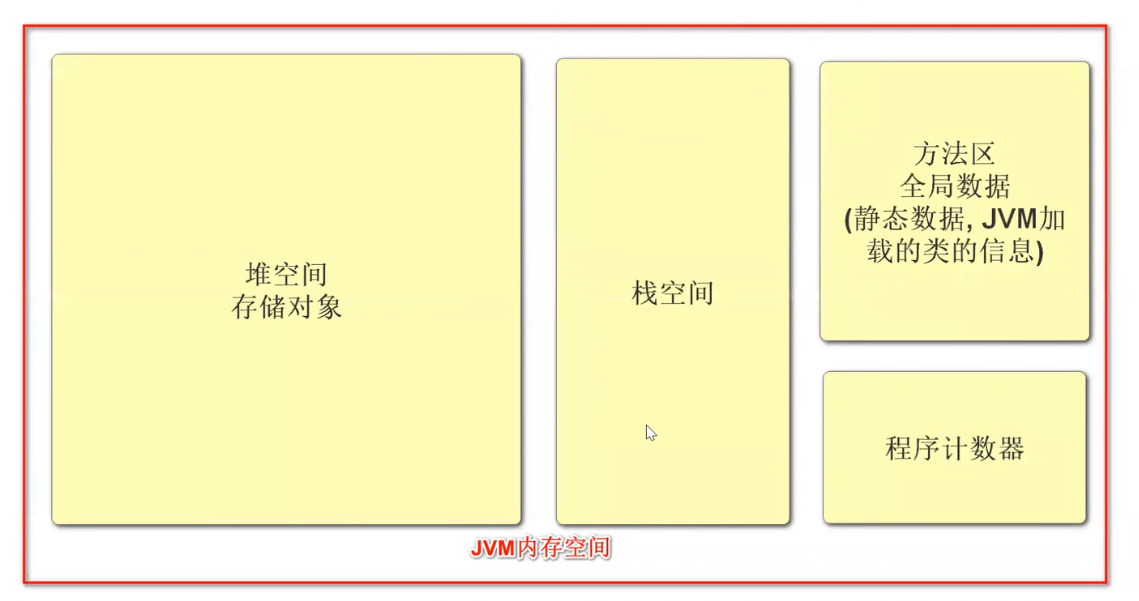``````闺中少妇不知愁     天泽职员几多愁

``````

## P23-函数的递归 1:10:42

### 阶乘函数

10:55 —20201227

``````//阶乘函数

package day5;
public class test0501{

public static void main(String[] args){

java.util.Scanner sc = new java.util.Scanner(System.in);
int n= sc.nextInt();
System.out.println(fact(n));
}
static int fact(int n){

// if(n==1) return 1;
int result = n* fact(n-1);
return result;
}
}

C:\workspace\workspace_idea\javaBasic\baizhi_hu>javac -d . *.java

C:\workspace\workspace_idea\javaBasic\baizhi_hu>java day5.test0501

``````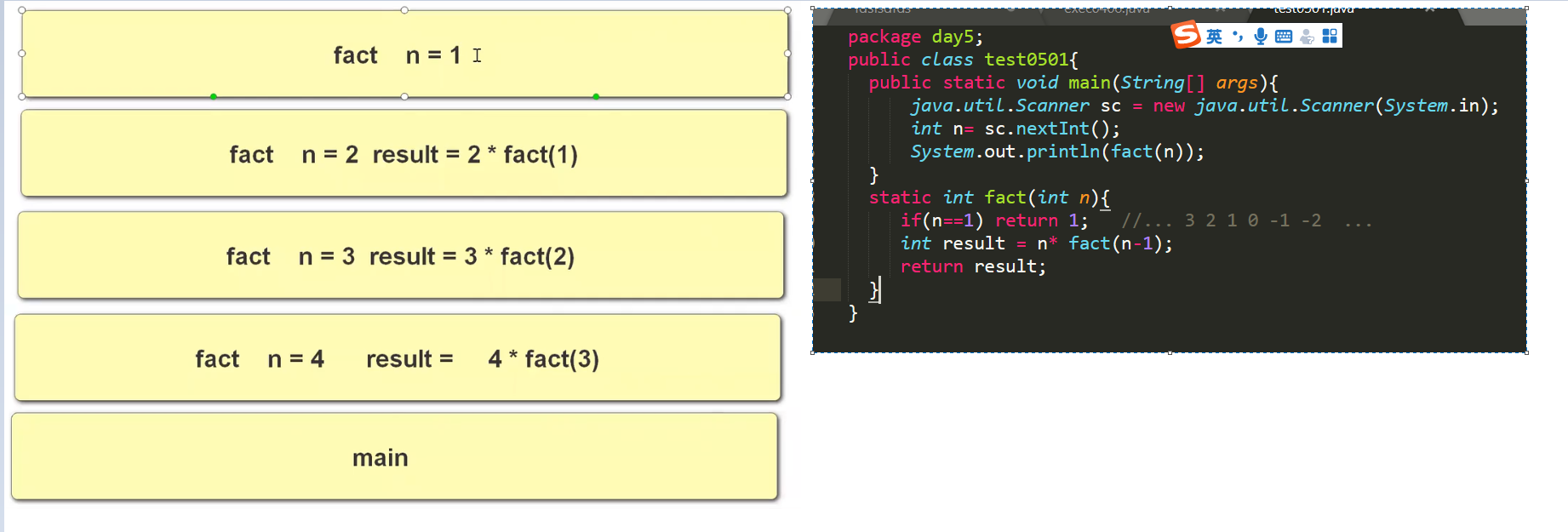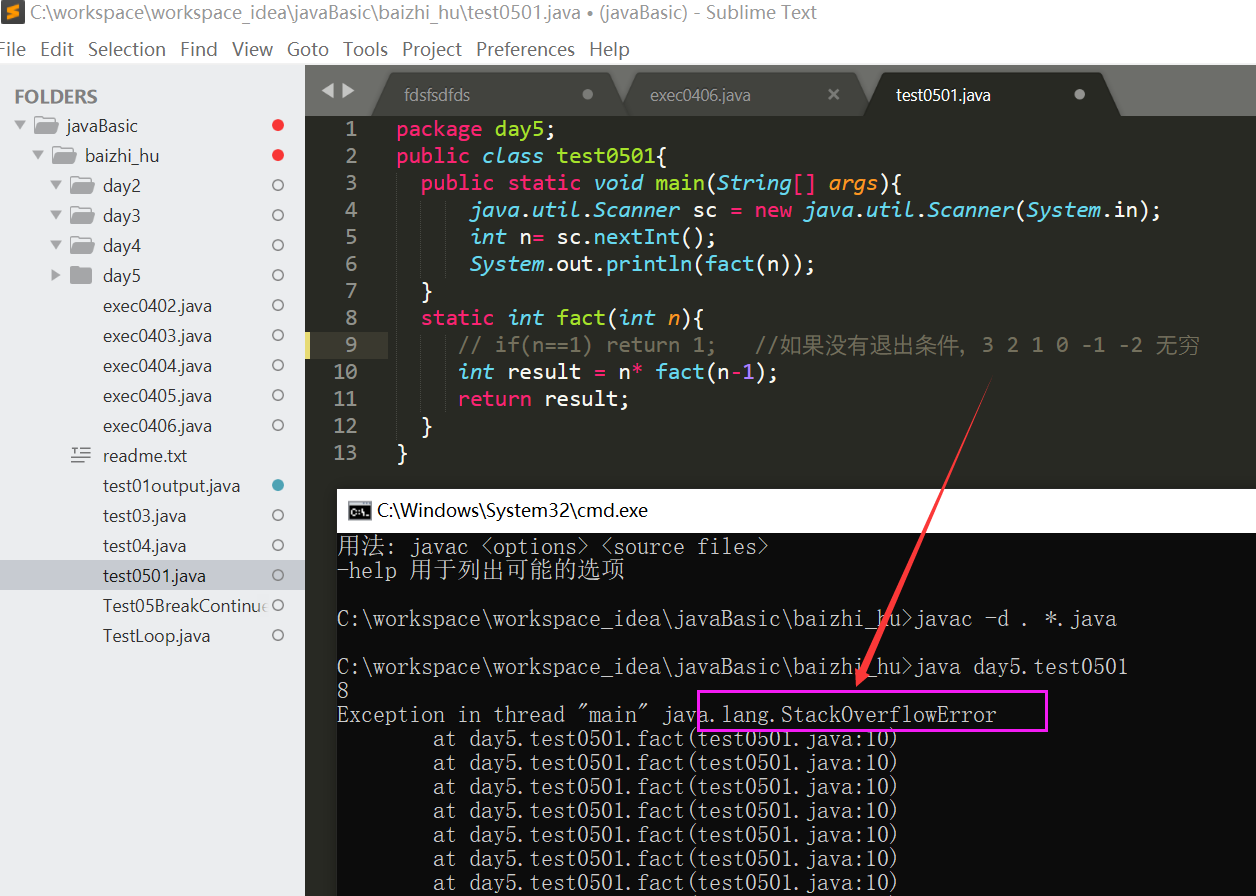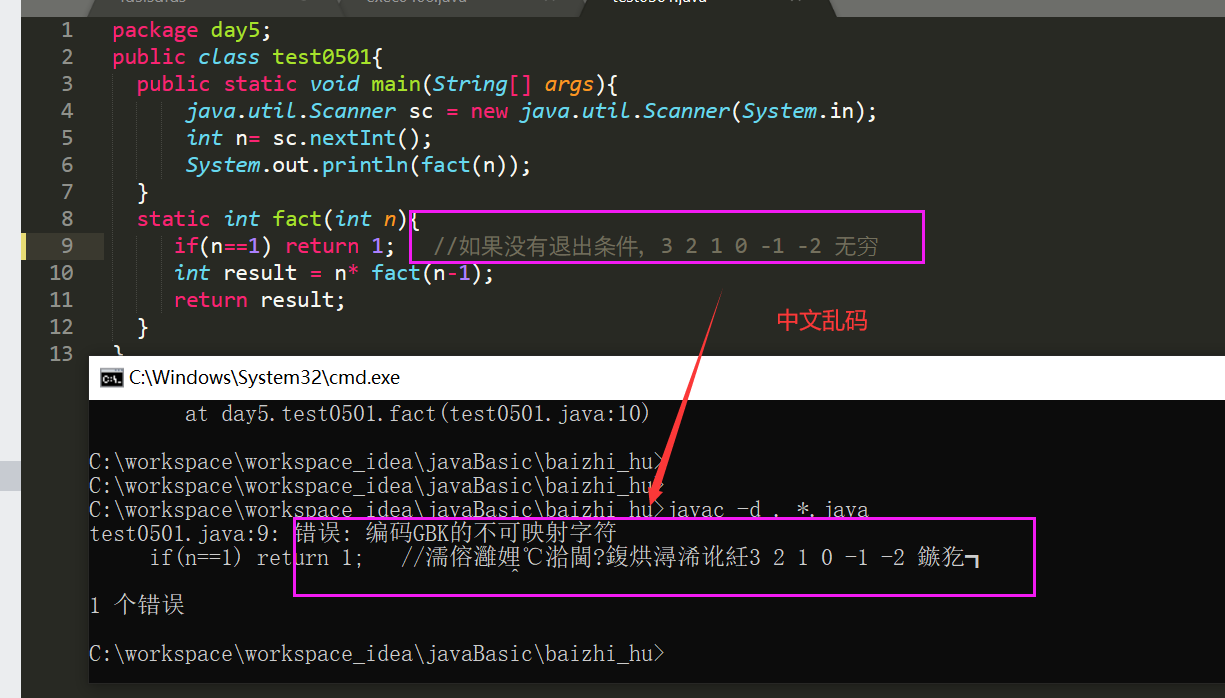### 斐波那契数列

``````//斐波那契数列  后一个数等于前两个数的和   test0502.java
//0 1 1 2 3 5 8 13

package day5;
public class test0502{

public static void main(String[] args){

java.util.Scanner sc = new java.util.Scanner(System.in);
int n= sc.nextInt();
System.out.println(fabo(n));
}
//calc   n-1  + n -2 = n
static int fabo(int n){

if(n==1) return 0;
if(n==2) return 1;
return fabo(n-1) + fabo(n-2);
}
}

``````

### 汉诺塔 hannota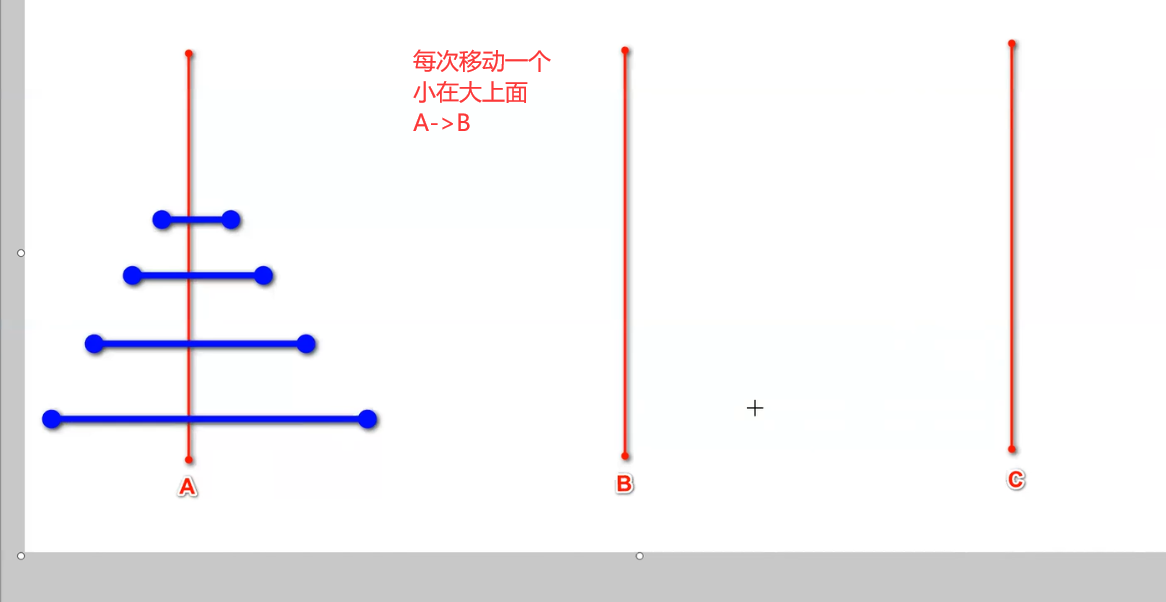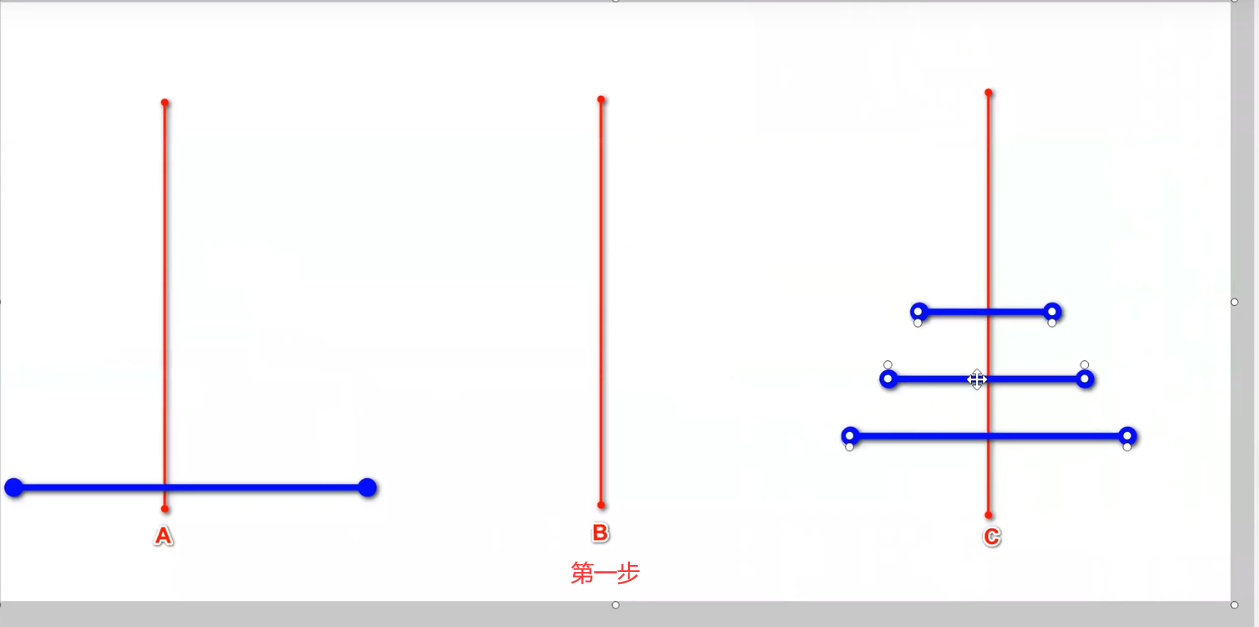第二步，把 最大的，从A移动到B

``````大问题要分解成很多的小问题， 小问题的解决方式通大问题相同

1. 如果A 只有两个盘子  小盘子放c , 大放B ,小盘子从C挪到B
2.

package day5;
//汉诺塔问题
public class test0503{

public static void main(String[] args){

java.util.Scanner sc = new java.util.Scanner(System.in);
int n= sc.nextInt();
/*
移动n个盘子  A -B
1.  移动 n-1 个盘子   A--> C
2.  将大盘子          A--> B
3.  移动 n-1 个判词   C --> B
-----------------------------到目前为止没有解决 ，因为不符合一次移动一个的条件
注意递归一定要有退出条件
*/
transfer(n,'A','B','C');
}
//把n 个盘子，从from移动到to ,借助temp
//static void transfer(int n,char A ,char B ,char C ){

static void transfer(int n,char from ,char to ,char temp ){

if(n==0) return ;
// 1.  移动 n-1 个盘子   from--> temp
transfer(n-1,from,temp,to);
// 2.  将大盘子          from--> to
System.out.println(from +"------->" + to );
// 3.  移动 n-1 个盘子   temp --> to
transfer(n-1,temp,to,from);
}
}

``````

## P24-函数习题课 30:43

``````C:\workspace\workspace_idea\javaBasic\baizhi_hu>javac -d . test0504.java
C:\workspace\workspace_idea\javaBasic\baizhi_hu>java  day5.test0504
1  3
// 传值 传引用问题
package day5;
// value reference
public class test0504{

public static void main(String[] args){

int m=1;int n=3;
exchange1(m,n);
System.out.println(m+ "  "+ n); // print 1 3 do not change
}
//
static void exchange1(int a,int b ){

int temp =a;
a=b;
b=temp;
}
}
``````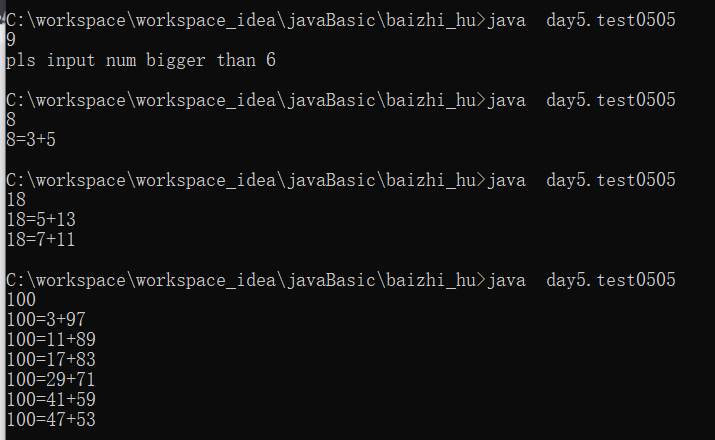``````package day5;
// 哥德巴赫猜想
// 任何一个大于6的偶数都能分解为两个质数的和，
// 要求，输入一个整数，输出 这两个被分解成的质数
public class test0505{

public static void main(String[] args){

java.util.Scanner sc =new java.util.Scanner(System.in);
int n=    sc.nextInt();
if(n<=6 || n%2==1){

System.out.println("pls input num bigger than 6 ");
return ;
}
/*
100=2+98
3+97
4+96
...
*/
for(int a=2;a<=n/2;a++){

int b=n-a;
// if a is zhishu  && b is zhishu
if(isPrime(a) && isPrime(b)){

System.out.println(n+"="+a+"+"+b);
}
}

}

//判断x是不是质数，如果是返回true
static boolean isPrime(int x){

for(int i=2;i<x;i++){

if(x%i==0) return false;
}
return true;
}

}
``````

``````package day5;
// 哥德巴赫猜想
// 任何一个大于6的偶数都能分解为两个质数的和，
// 要求，输入一个整数，输出 这两个被分解成的质数
public class test0506{

public static void main(String[] args){

java.util.Scanner sc =new java.util.Scanner(System.in);
int n=    sc.nextInt();
if(n<=6 || n%2==1){

System.out.println("pls input num bigger than 6 ");
return ;
}
/*
100=2+98
3+97
4+96
...
*/
//for(int a=2;a<=n/2;a++){

for(int a=3;a<=n/2;a+=2){
//update   01

int b=n-a;
// if a is zhishu  && b is zhishu
if(isPrime(a) && isPrime(b)){

System.out.println(n+"="+a+"+"+b);
}
}

}

//判断x是不是质数，如果是返回true
static boolean isPrime(int x){

double d = Math.sqrt(x);
for(int i=3;i<d;i+=2){
//update 02  because  100   2*50   4*25  5*20  10*10
//for(int i=3;i<x;i+=2){
//update   01
//for(int i=2;i<x;i++){

if(x%i==0) return false;
}
return true;
}

}
``````

# part5 数组

## P25-数组的基本语法 37:42

``````一次性定义多个相同类型的变量，方便管理，访问
1. 定义数组
int[] a;
int []a;
int a[];   //定义数组三种方式，通常用第一种方式
2.为数组分配空间
a = new int;           //
a = new int[] {
1,3,6,8};  //显式初始化

int[] a = new int[]{
1,3,5,7,8};    //完整写法
int[] a ={
1,3,5,7,9};              //简略写法
3.数组默认值
0  false  null
0 ,各种形式的0  基本数据类型 7/8 （byte short int long float double char ）
false, boolean 基本数据类型 1/8
null, 对象数据类型默认值

``````
``````package day5;
public class test0507arr{

public static void main (String[] args){

int[] a;   //multial types of  int
a=new int;
//
System.out.println("---------1---------");
for(int i=0;i<5;i++){

System.out.println(a[i]);
}
//defalut is 0  0 0 0 0
System.out.println("---------2---------");
for(int i=0;i<5;i++){

a[i]=i+1;
}
//System.out.println(a.length);
//System.out.println(a.toString());  //[I@659e0bfd
System.out.println(a);
System.out.println("---------3--------");
}
}
``````

## P26-数组的内存结构 1:19:44

int[] a=new int; //数组是个对象 ，存放在堆空间中
// new —> 表示在堆空间分配内存地址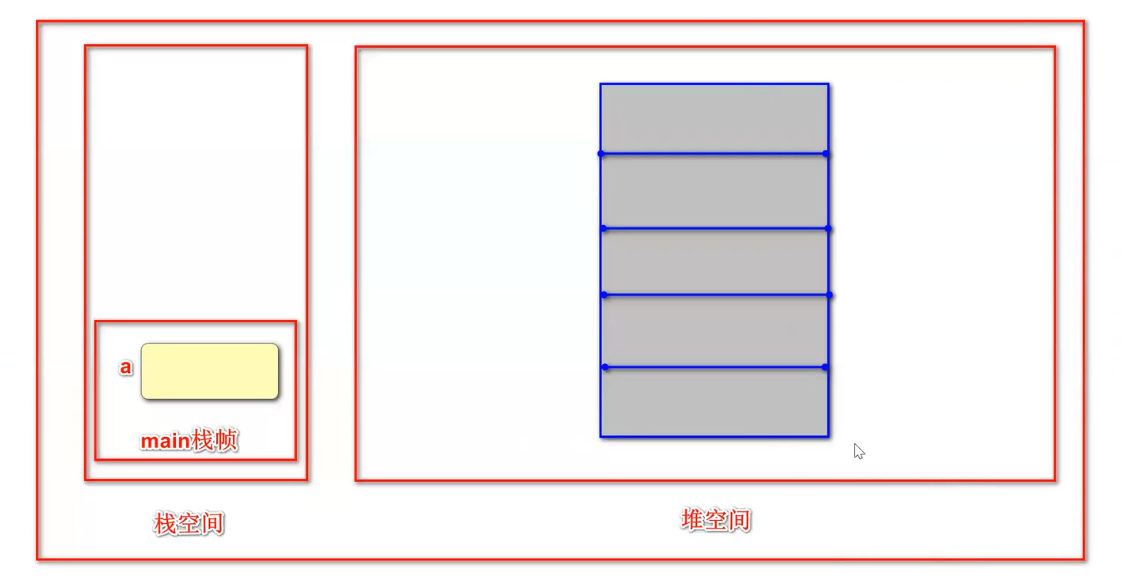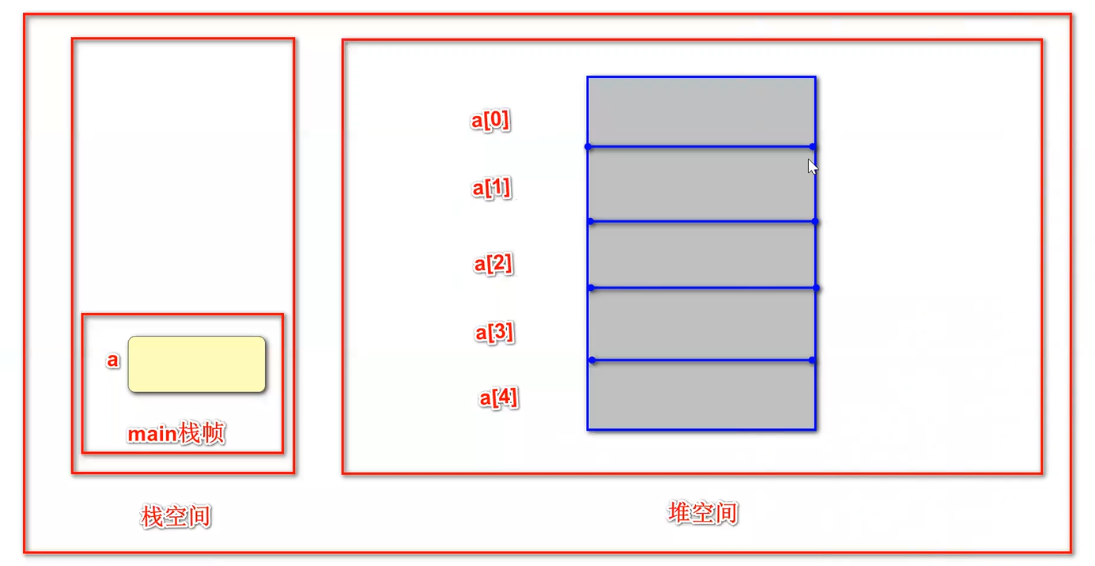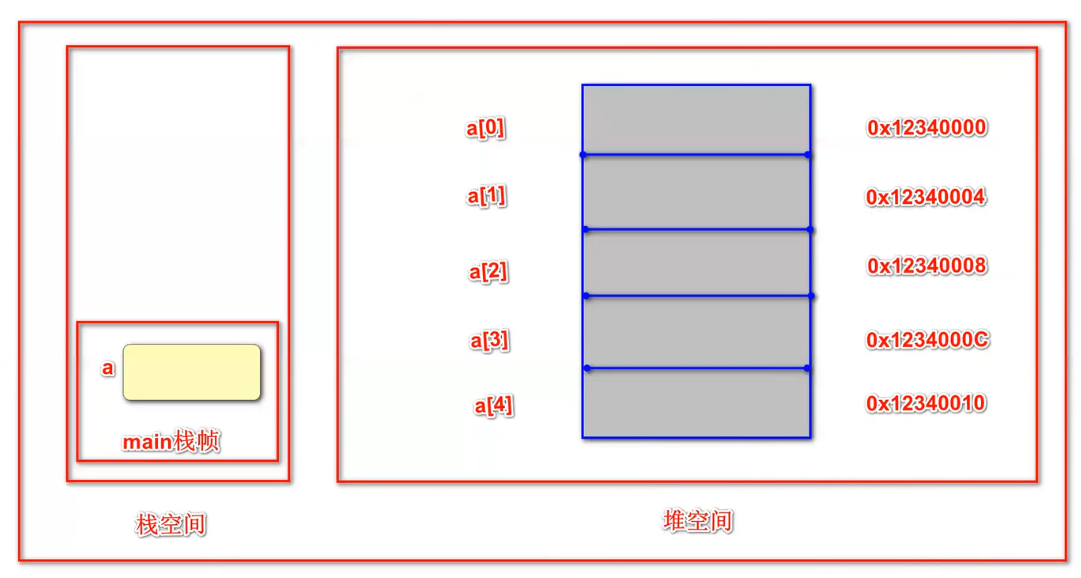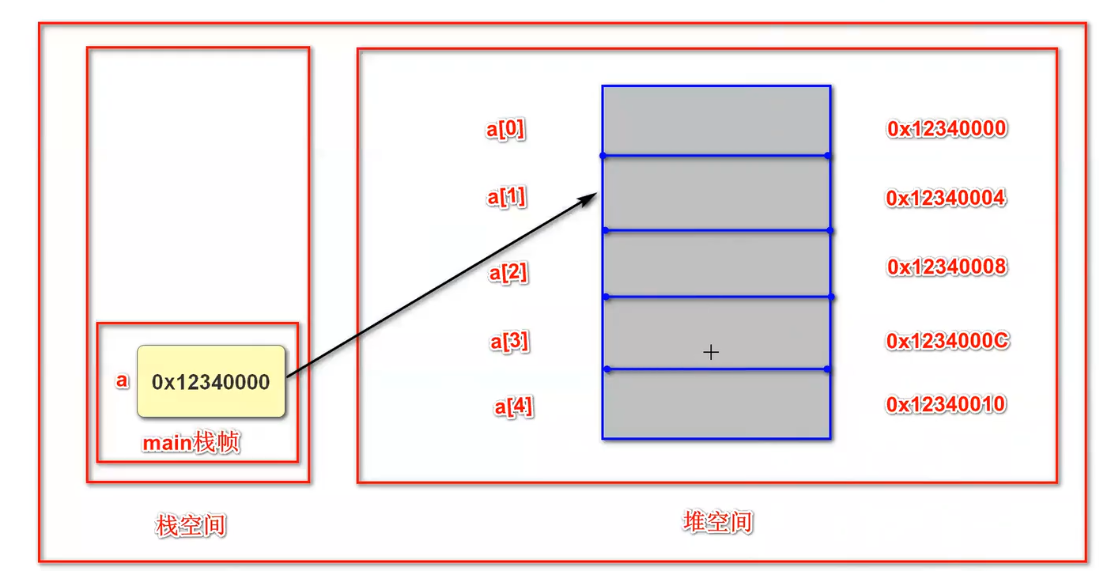``````上图可知
1.数组空间在内存中是连续的
数组的长度固定，不可改变，见上图，理解它
数组下标从0开始， a[n]=a的首地址+n*4
数组结构查询效率最高，添加删除元素效率最低（查询快，增删慢）
扩展，数组  <------->  链表  结构对应
链表结构查询效率低，添加删除元素效率高
链表如果要查询某个值，比如a8,需要从 a1中 找a2中 找 a3中 找a4 ... a7 中找  a8
2.数组变量存储的是数组地址
``````

#### 我换媳妇了 a=b;

``````       int[] a ={
1,2,3,4,5};
int[] b =new int[a.length*2];
a=b;  // 把b变量的值赋值给a
``````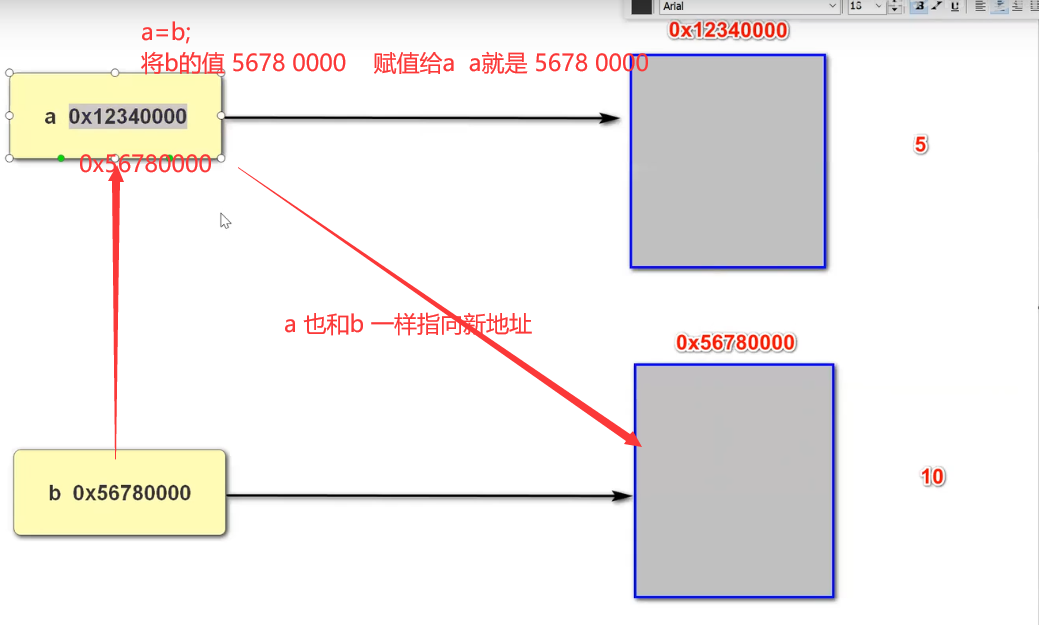``````  int[] a ={
1,2,3,4,5};
/*  // 数组长度扩张方式1
int[] b =new int[a.length*2];
for(int i=0;i<a.length;i++){

b[i]=a[i];
}
*/
//   // 数组长度扩张方式2
int[] b = java.util.Arrays.copyOf(a,a.length*2);

System.out.println(a.length);
a=b;
System.out.println(a.length);
for(int i=0;i<a.length;i++){

System.out.println(a[i]);
}
``````

#### 传引用示例2

``````int[] a={
1,2,3,4,5};
int[] b=a;
b= 22;
System.out.println(a);
//a  b  都是10 ，因为 a b 放到是堆的地址，
``````

#### 传引用示例3

``````public static void main(String[] args){

int[] a={
1,2,3,4,5};
change(a);
System.out.println(a);
}

static void change(int[] a){

a=10;
}
``````

## P27-二维数组 29:45

``````  int[][] a;
a=new int;
/*
a  a  a  a
a  a  a  a
a  a  a  a
*/
``````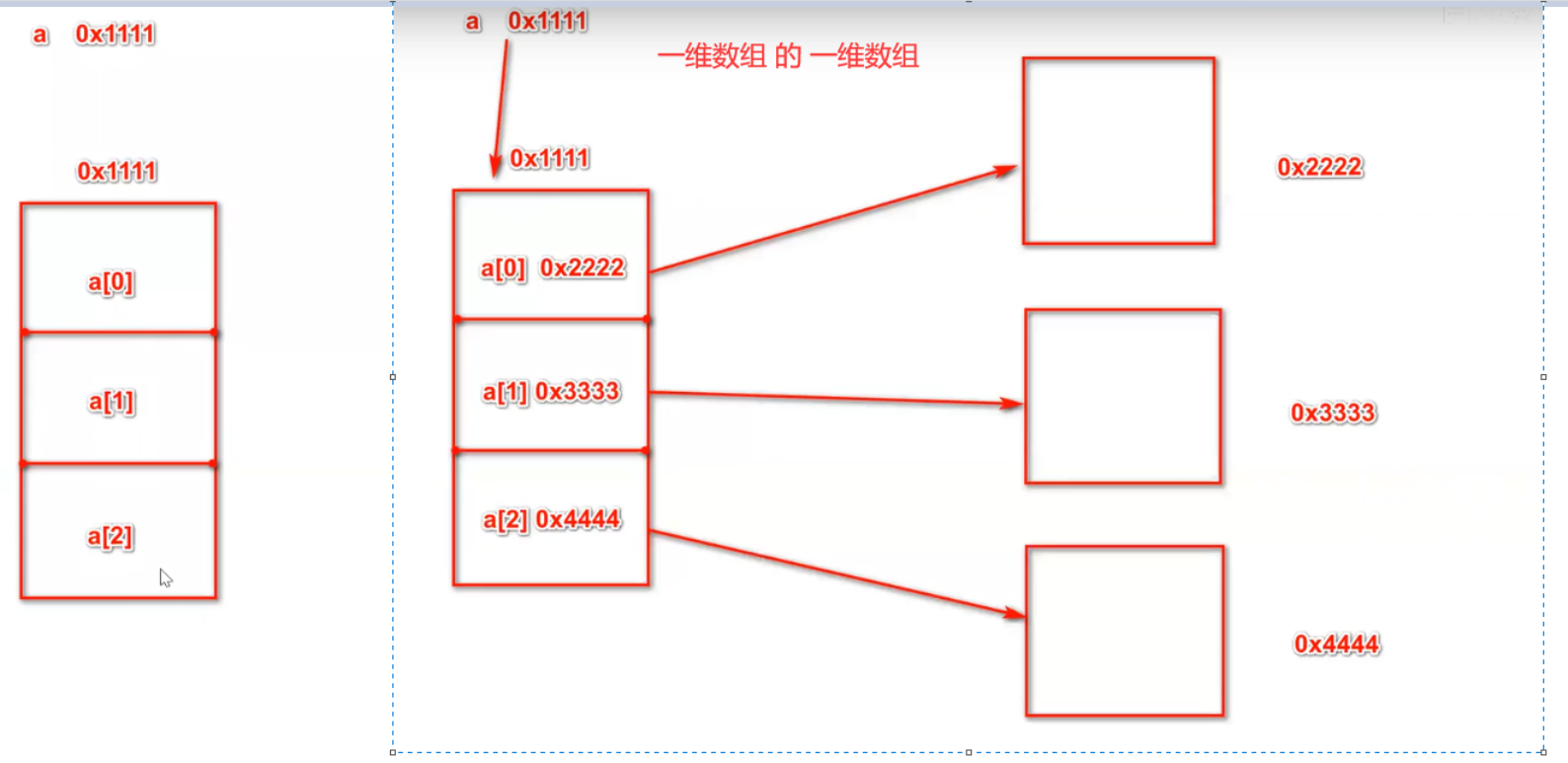``````package day5;
public class test0509arr{

public static void main (String[] args){

//type 01
//int[][] a;       a=new int;
//type 02
//int[][] a ={
{
1,2,3,4},{
5,6,7,8},{
1,3,5,7}};
// type 03
//int[][] a ={
{
1,2,3,4,5},{
5,6,7},{
1,3,5,7}};
//type 04
int[][] a =new int[];   //it is ok
a =new int;
a =new int;
a =new int;

/*
a  a  a  a
a  a  a  a
a  a  a  a
*/
for(int i=0;i<a.length;i++){

for(int j=0;j<a[i].length;j++){

System.out.print(a[i][j]+"\t");
}
System.out.println();
}

System.out.println("---------1---------");

//defalut is 0  0 0 0 0
System.out.println("---------2---------");

System.out.println("---------3--------");
}
}
``````

## P28-排序算法 44:18

### 冒泡排序

``````package day6;
public class test0601order{

public static void main (String[] args){

//
int[] a = {
8,6,1,4,5,7};
int n =a.length;

System.out.println("origion is : ");
for(int k=0; k< n;k++){

System.out.print(a[k]+"\t");
}
System.out.println();

for(int i=0;i<n-1;i++){

for(int j=0;j<n-1-i;j++){

if(a[j]>a[j+1]){

int tmp = a[j];
a[j]=a[j+1];
a[j+1]=tmp;
}
}
}

System.out.println("order result is :");
for(int k=0; k< n;k++){

System.out.print(a[k]+"\t");
}
System.out.println();

}
}

C:\workspace\workspace_idea\javaBasic\baizhi_hu>java day6.test0601order
origion is :
8       6       1       4       5       7
order result is :
1       4       5       6       7       8
``````

### 选择排序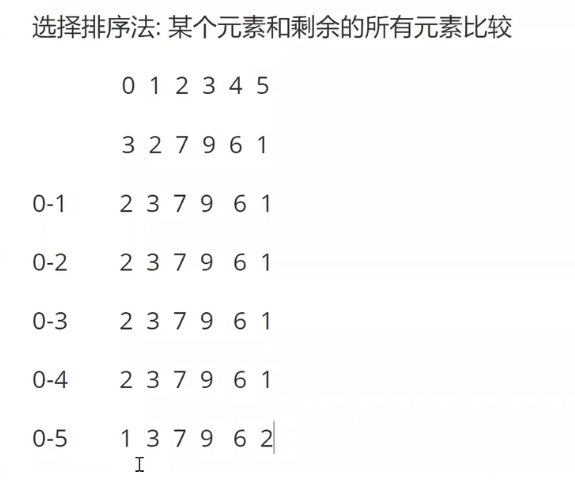``````C:\workspace\workspace_idea\javaBasic\baizhi_hu>javac -d . test0602order.java

C:\workspace\workspace_idea\javaBasic\baizhi_hu>java day6.test0602order
origion is :
8       6       1       4       5       7
order result is :
1       4       5       6       7       8
``````
``````package day6;
public class test0602order{

public static void main (String[] args){

//
int[] a = {
8,6,1,4,5,7};
int n =a.length;

System.out.println("origion is : ");
for(int k=0; k< n;k++){

System.out.print(a[k]+"\t");
}
System.out.println();

for(int i=0;i<n;i++){

for(int j=i+1;j<n;j++){

if(a[i]> a[j]){

int tmp =a[i];
a[i]=a[j];
a[j]=tmp;
}
}

}

System.out.println("order result is :");
for(int k=0; k< n;k++){

System.out.print(a[k]+"\t");
}
System.out.println();

}
}
``````

### jdk 排序法

java.util.Arrays.sort(a);

## P29-数组习题课-1 18:37

``````C:\workspace\workspace_idea\javaBasic\baizhi_hu>java day6.test0603
4
ddcd
``````
``````package day6;
public class test0603{

public static void main (String[] args){

char[] cs={
'A','d','G','d','F','c','d'};
char[] csResult=new char[cs.length];
int result=0;
for(int i=0;i<cs.length;i++){

if(cs[i]>='a' && cs[i]<='z')
{

csResult[result]=cs[i];
result ++;
}
}
System.out.println(result);
System.out.println();
for(int i=0;i<csResult.length;i++){

System.out.print(csResult[i]);
}
}
}
``````

## P30-数组习题课-2 1:29:20

### 八皇后问题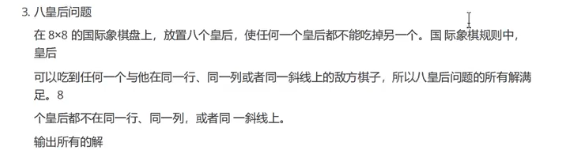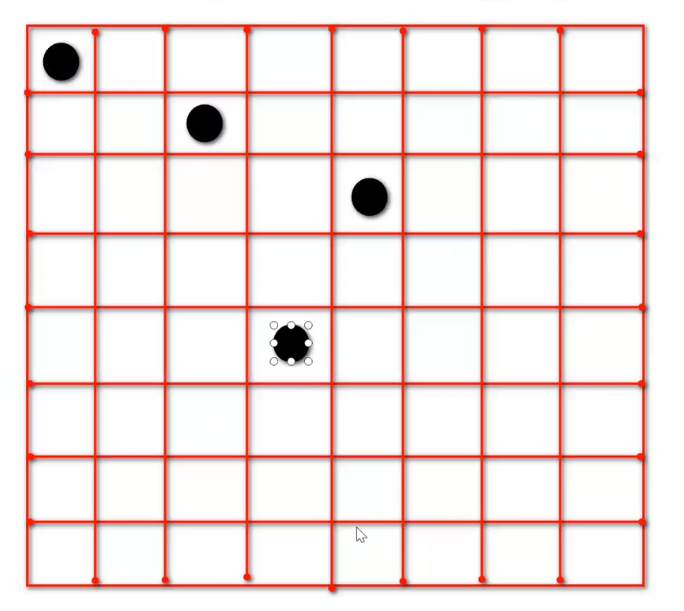``````在这里插入代码片
``````

### 找出单独的数据 使用异或非常快

``````package day6;
public class test0606{

public static void main (String[] args){

// single data
int[] a = {
2,5,4,8,7,5,8,4,7};
int result=0;
for(int i=0; i<a.length; i++){

result = result ^ a[i] ;
}
System.out.println(result);
}
}
``````

### 八皇后问题

``````package day6;
public class test0608queens{

// a[i]
static int[] a =new int;

public static void main (String[] args){

place(0);

}
/*
*/
static void place( int  n ){

if(n==8){

for(int i=0;i<a.length;i++){

System.out.print(a[i]+ "\t");
}
System.out.println();
return ;
}
//
outer: for(a[n]=0;a[n]<=7;a[n]++){

for(int j =0; j< n; j++){

if(a[j]==a[n] ||   a[j]-a[n]==j-n  ||  a[j]-a[n]==n-j  ){

//
continue outer;
}

}
//
place(n+1);

}

}

}
``````

``````package day6;
public class test0608queens{

// a[i]  第i行的皇后，放在第a[i]列
static int[] a =new int;

public static void main (String[] args){

place(0);

}
/* 放置第i行的皇后
尝试第 0 -7 列
如果和前面的皇后有冲突，尝试下一列
如果和前面的皇后没有冲突，放置第i+1行的皇后
*/
static void place( int  n ){

if(n==8){

for(int i=0;i<a.length;i++){

System.out.print(a[i]+ "\t");
}
System.out.println();
return ;
}
//尝试第 0 -7 列
outer: for(a[n]=0;a[n]<=7;a[n]++){

for(int j =0; j< n; j++){

//判断a[j] 和 a[n] 是否有冲突
if(a[j]==a[n] || a[j]-a[n]==j-n || a[j]-a[n]==n-j ){

//行差 == 列差 说明在斜线上面
continue outer;
}
}
//如果和前面的皇后都没有冲突，放置第n+i行的皇后
place(n+1);

}

}

}
``````

### MatLab实现均值滤波器_matlab conv2用于均值滤波-程序员宅基地

% 3*3 均值滤波kernel_size = [3,3];kernel = ones(kernel_size)/(kernel_size(1)*kernel_size(2));padding_size = (kernel_size-1)/2;img_height = size(I2,1);img_width = size(I2,2);img_channels = size(I2,3);..._matlab conv2用于均值滤波

### Spring学习记录01_@around(value = pointcut_goods_update) public obje-程序员宅基地

JavaEE概述第一阶段：Java2.0 版本提出Java划分为3大平台;JavaSE：标准版 （桌面应用：eclipse ，Navicat，IDEA）JavaME：微型版 （移动端应用）JavaEE：企业版 （开发大型企业应用）大型企业应用需求1、高并发（能够支持大量的用户同时在线 几万-几百万）；2、大数据量（单表的数据可能都达到 几千万）；3、支持分布式事务；4、支持集群，支持负载均衡；注意：单台的tomcat 在单位时间1s内，能支持0-500个并发请求；需要的技术轻量级框架_@around(value = pointcut_goods_update) public object cachegoodsupdate(procee